## CPSC 110-08: Computing on Mobile Phones Spring 2012 How to Flip A Coin, Part I

### CS Principles

This activity addresses the concepts of abstraction. It provides a simple illustration of how simulation and modeling are used in computing. It focuses, in part, on the following learning objectives:
• 5: The student can describe the combination of abstractions used to represent data.
• 9: The student can use models and simulations to raise and answer questions

### Preliminaries

In this tutorial we'll use App Inventor's random number generator to simulate a coin flip. Randomness is an important element in many computer applications, especially games and simulations. If you are developing some kind of game for your first creative project, you will probably want to incorporate randomness into the app.

### Pseudo Random Number Generators

A truly random event is completely unpredictable.

• Pick 1 of 25 numbered marbles out of a completely mixed up jar of marbles. (1 in 25 chance of picking a particular number).

• Flip a fair coin: 1 in 2 chance of it coming up heads.

• Pick a card out of a thoroughly shuffled deck (4 in 52 chance of drawing an Ace).

A pseudo random event looks random but is completely predictable (deterministic). This is the kind of randomness used by most computer games.

A pseudo random number generator (PRNG) is a mathematical function that generates a random-looking sequence.

PRNGs are useful in:

• Cryptography -- used to generate secret keys.

• Computer games -- card games, shooter games, adventure games, etc.

• Simulation -- weather models, physical systems, etc.

For example, here's an formula for generating a random-looking sequence of numbers:

Xn+1 = (aXn + b) mod m

This formula says, to generate Xn+1, the n+1st number in a sequence, multiply Xn, the ntn number in the sequence, by a then add b and then take the remainder after dividing that quantity by m.

(Are you familiar with the mod operator? This is the operator, represented by the remainder block in App Inventor's Math drawer, that takes the remainder of an integer division operation. For example, in 5 / 2 the quotient is 2 and the remainder is 1. Thus, 5 mod 2 equals 1.)

This process of generating the n+1st number from the nth number will generate a random-like sequence of numbers between 0 and m-1. The sequence will appear random, but if you know the nth number, you can predict the n+1st number. And if you know the first number, X0, called the seed, you can predict every number in the sequence (given the constants a and b).

For example, if we let a = 5, b = 3, m = 11 and X0 = 10, we get the following sequence of numbers: 10, 9, 4, 1, 8, 10, ,,,

a  b  m  Xi Calculation Xi+1
531110(5 × 10 + 3) mod 11 = 53 mod 11 = 9
53119(5 × 9 + 3) mod 11 = 48 mod 11 =4
53114(5 × 4 + 3) mod 11 = 23 mod 11 =1
53111(5 × 1 + 3) mod 11 = 8 mod 11 =8
53118(5 × 8 + 3) mod 11 = 43 mod 11 =10

This isn't a particularly good sequence because it only contains the numbers 10, 9, 4, 1, 8. Mathematicians who study PRNGs try to find values for a, b and m that generate long, repeating sequences.

### Randomness in App Inventor

You can find the random functions in the Blocks Editor under the Math menu, where you find:

 random fraction -- returns a random value between 0 and 1 random integer -- returns a random integer between its from and to arguments, inclusive random set seed -- sets the seed for the PRNG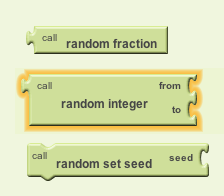### The CoinFlipper App -- Version 1

Version 1 of our app will use two coin Images, one for heads and one for tails. Click on these to download them to your computer:

Our app's user interface will consist of three App Inventor components:

• A Button that the use can click to "flip the coin."
• An Image that will display either "heads" or "tails"
• A TextToSpeech component (Other Stuff drawer) that will say "heads" or "tails"

Drag and drop each of these components onto your app and configure it as shown in this screenshot (click to enlarge):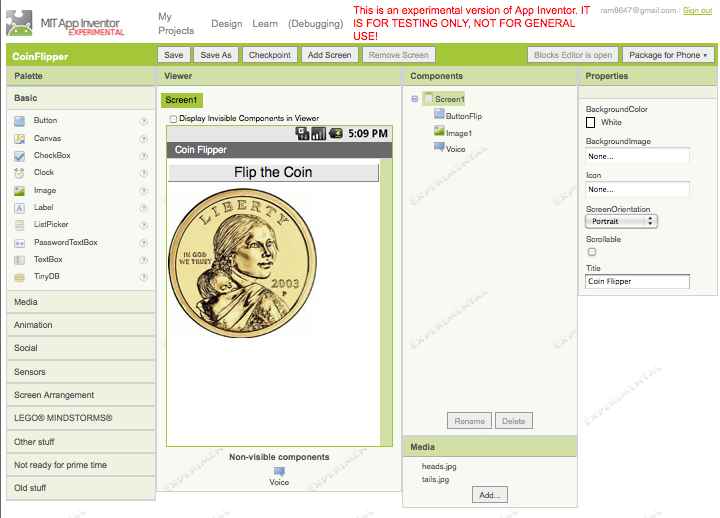Notice that TextToSpeech component is a non-visible component.

This completes the User Interface portion of the app.

#### Algorithm and Data Abstraction

Let's now program the app's behavior in the Blocks Editor. Our Data Abstraction: In this app we will use a global variable, named Coin, to represent the coin's current state (heads or tails). We will assume that heads is represented as 1 and tails is represented as 2. So Coins=1 means heads and Coins=2 means tails: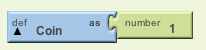Our Algorithm: Our algorithm will use an if/else control structure to switch randomly between heads and tails. It can be describe in pseudocode as follows. First we set Coin's value randomly to 1 or 2 and then we display either heads or tails to the user:

```When the ButtonFlip is Clicked do:
Set Coin to either 1 or 2 selected randomly
If Coin = 1 then-do:
else-do:
Say "Tails"
Display the Tails Image
```

To "flip the coin" we will modify the coin's current state (heads or tails) by setting Coin's value to 1 or 2 using App Inventor's random integer function (in the Math drawer).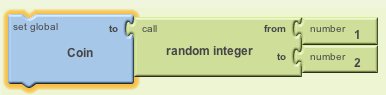To display the coin's current state, we'll use an if/else block (in the Control drawer):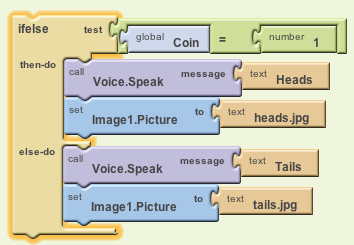All of these actions will take place whenever the user click's the ButtonFlip button. Here's how all this would be implemented in App Inventor (click to enlarge):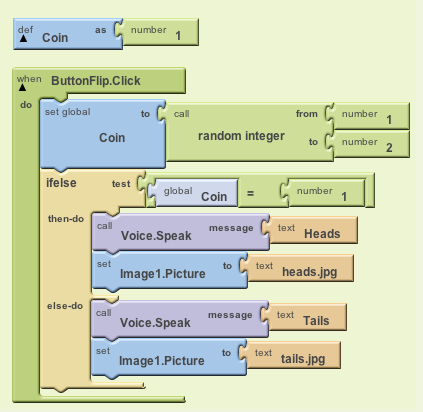### Testing

Test your app and work out all the bugs. Every time you click the button, it should display and speak the current state of the coin (either heads or tails).

### Homework Handin

Create a page for this assignment under the Homework tab on your Portfolio. The page should contain:

• A description of this tutorial in your own words.